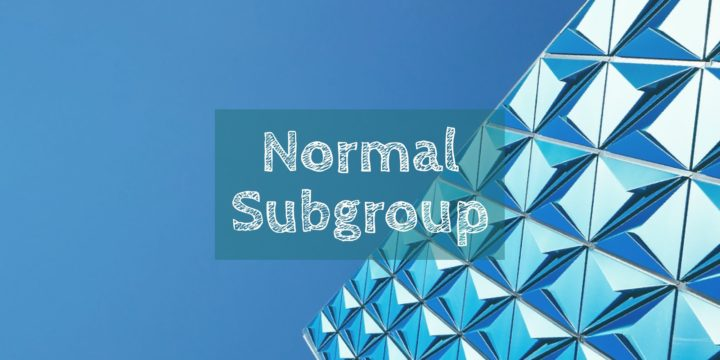# Normal Subgroups Intersecting Trivially Commute in a Group## Problem 49

Let $A$ and $B$ be normal subgroups of a group $G$. Suppose $A\cap B=\{e\}$, where $e$ is the unit element of the group $G$.
Show that for any $a \in A$ and $b \in B$ we have $ab=ba$.Add to solve later

Contents

## Hint.

Consider the commutator of $a$ and $b$, that is, $aba^{-1}b^{-1}$.

## Solution.

Consider the product $aba^{-1}b^{-1}$. Since $A$ is normal in $G$, the element $ba^{-1}b^{-1} \in A$ as it is the conjugate of $a^{-1}\in A$.
Thus $aba^{-1}b^{-1}=a(ba^{-1}b^{-1} ) \in A$.

Similarly, since $B$ is normal in $G$, we have $aba^{-1} \in B$.

Hence $aba^{-1}b^{-1}=(aba^{-1})b^{-1} \in B$.
Therefore $aba^{-1}b^{-1} \in A\cap B=\{e\}$ and we see that $aba^{-1}b^{-1}=e$, thus $ab=ba$.Add to solve later

### More from my site

#### You may also like...

This site uses Akismet to reduce spam. Learn how your comment data is processed.

###### More in Group Theory##### The Center of the Symmetric group is Trivial if $n>2$

Show that the center $Z(S_n)$ of the symmetric group with $n \geq 3$ is trivial.

Close